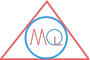# Vertices, Edges, Faces of 3-D Shapes & Euler’s Formula

## Table of vertices, edges and faces of 3-D shapes

ShapeNo. of verticesNo. of edgesNo. of faces
Cube8126
Cuboid8126
Cylinder023
Sphere001
Cone112
Prism695
Triangular pyramid464

## Euler’s formula

Euler’s formula is used to find number of faces or number of vertices or number of edges.

Formula

Euler’s formula
$$F + V = E + 2$$

Example

So, let’s validate Euler’s formula for cube:
V = 8, E = 12, F = 6
Using Euler’s formula, F + V = E + 2
$$\therefore$$, 6 + 8 = 12 + 2
14 = 14